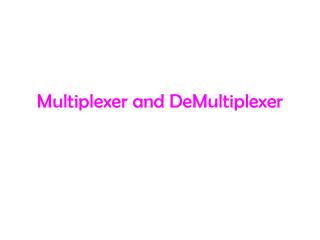DownloadDownload PresentationMultiplexer and DeMultiplexer

# Multiplexer and DeMultiplexer

Download Presentation## Multiplexer and DeMultiplexer

- - - - - - - - - - - - - - - - - - - - - - - - - - - E N D - - - - - - - - - - - - - - - - - - - - - - - - - - -
##### Presentation Transcript

1. Multiplexer and DeMultiplexer

2. Multiplexer (Data Selectors) • The Term ‘Multiplex’ means “many into one”. • Multiplexing is the process of transmitting a large number of information over a single line.

3. Cont., • A digital multiplexer (MUX) is a combinational circuit that selects one digital information several sources and transmits the selected information on a single output line.

4. Cont., • A multiplexer is also called a data sector • The multiplexer has several data-input lines and a single output line.

5. Block diagram of Multiplexer

6. 4 to 1 Multiplexer Logic Symbol S1 Select inputs S0 Y 4-to-1 MUX D0 D1 Data inputs Data output D2 D3

7. 4 to 1 Multiplexer Logic diagram

8. Cont., • To demonstrate the operation of the circuit, consider the case when S1 S0 =00. • If S1 S0 =00 is applied to the select lines, the AND gate associated with D0 will have two of its input equal to 1 and the third input connected to D0. • The other three AND gates have 0 in at least one of their inputs, which make their output equal to 0. • Hence, the OR output (Y) is equal to the value of D0.

9. Cont., • Thus, it provides a path from the selected input and the data on the input D0 appears on the data-output line (Y). • If S1 S0 =01 (binary 1) applied to the select lines , the data on the input D1 appears on the data output line.

10. Cont., • If S1 S0 =10 (binary 2) is applied, the data on the input D2 appears on the output line (Y). • Similarly, if S1 S0 =11 is applied, the data on D3 is switched to the output line (Y). • The AND gates and the inverters resemble a decoder circuit, and indeed they decode the input select lines. • In general, a 2n–to-1 multiplexer is constructed from n-to -2n decoder by adding to it 2n input lines, one each AND gate.

11. Cont., • The output of the AND gates are applied to a single OR gate to provide a single output. • The size of the multiplexer is specified by the number 2n of input lines and the single output line. • Multiplexer ICs have an enable input to control the operation of the unit. • The enable input (also called strobe) can be used to cascade two or more multiplexer ICs to construct a multiplexer with larger number of inputs

12. Truth table of 4-to-1 Multiplexer

13. DeMultiplexer (Data Distributors) • The word “Demultiplex” means “One into many”. • DeMultiplexing is the process of taking information from one input and transmitting the same over one of several outputs.

14. Block diagram of DeMultiplexer Output 0 Output 1 Input Output 2 Output 3 S1 S0 (Select pins)

15. 1 to 4 DeMultiplexer Logic Symbol S1 Y0 Select inputs S0 Y1 1-to4 DMUX Y2 Data input D Y3 Data outputs

16. 1 to 4 DeMultiplexer Logic diagram

17. Cont., • A 1-to-4 DeMultiplexer has a single input (D), four outputs (Y0 to Y3) and two select inputs (S0 and S1).

18. Truth Table of 1 to 4 DeMultiplexer

19. Cont., • From the truth table, it is clear that the data input is connected to output Y0 when S1=0 and S0=0 and the data input is connected to output Y1 when S1=0 and S0=1. • Similarly, the data input is connected to outputs Y2 and Y3 when S1=1 and S0=0 and when S1=1 and S0=1 respectively.

20. Expressions of output Y0 = S1 S0 D Y1 = S1 S0 D Y1 = S1 S0 D Y1 = S1 S0 D

21. Cont., • Now, using the above expressions, a 1-to-4 DeMultiplexer can be implemented using four 3- input AND gates and two NOT gates. • Here, the input data line is connected to all the AND gates

22. Cont., • The two select lines S1 and S0 enable only one at a time and the data that appears on the input line passes through the selected gate to the associated output line.

23. The End ….. Thank you ….. M.S.P.V.L. Polytechnic college, Pavoorchatram.